Try NerdPal! Our new app on iOS and Android

# Factor the expression $x^2-2x+1$

## Related Videos

Go!
Go!
1
2
3
4
5
6
7
8
9
0
a
b
c
d
f
g
m
n
u
v
w
x
y
z
.
(◻)
+
-
×
◻/◻
/
÷
2

e
π
ln
log
log
lim
d/dx
Dx
|◻|
θ
=
>
<
>=
<=
sin
cos
tan
cot
sec
csc

asin
acos
atan
acot
asec
acsc

sinh
cosh
tanh
coth
sech
csch

asinh
acosh
atanh
acoth
asech
acsch

### Videos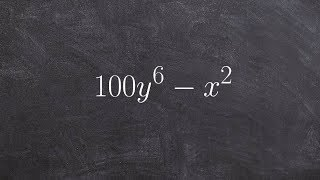### Algebra 1 - Factor difference of two squares - The best math teacher ever 100y^6 - x^2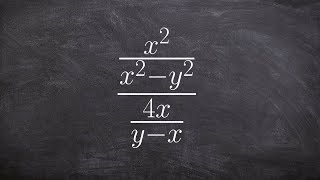### Algebra 2 - Dividing two rational expressions (x^2/x^2 - y^2) / (4x/y-x)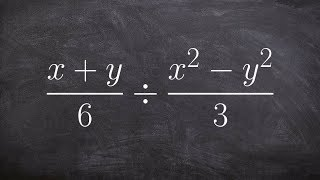### Algebra 2 - Dividing two rational expressions by factoring, ((x + y)/6) / ((x^2 - y^2)/3)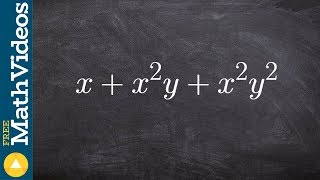### Algebra 1 - How to factor the GCF from a trinomial x+ x^2y + x^2 y^2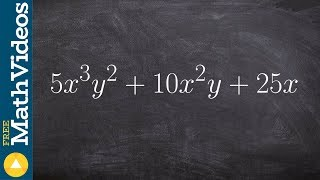### Algebra 1 - Factoring three terms with distributive property 5x^3 y^2 + 10x^2y + 25x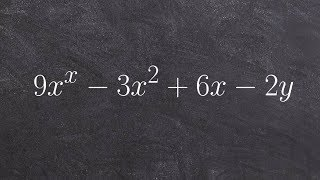SnapXam A2

### beta Got another answer? Verify it!

Go!
1
2
3
4
5
6
7
8
9
0
a
b
c
d
f
g
m
n
u
v
w
x
y
z
.
(◻)
+
-
×
◻/◻
/
÷
2

e
π
ln
log
log
lim
d/dx
Dx
|◻|
θ
=
>
<
>=
<=
sin
cos
tan
cot
sec
csc

asin
acos
atan
acot
asec
acsc

sinh
cosh
tanh
coth
sech
csch

asinh
acosh
atanh
acoth
asech
acsch

$factor\left(x^2-2x+1\right)$